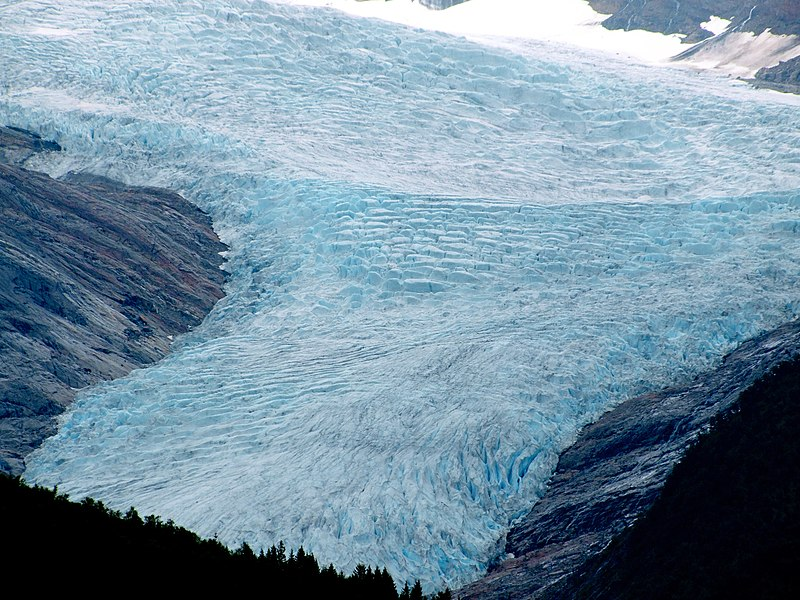# Mass Balance# Mass Balance

## What is glacier mass balance?

The Mass balance of a glacier can be thought of as the health of a glacier. Mass balance is the total sum of all the accumulation (snow, ice, freezing rain) and melt or ice loss (from calving icebergs, melting, sublimation) across the entire glacier.

If glaciers have a mass balance that is in equilibrium with climate, then the inputs are equal to the outputs. The glacier remains the same size, and does not grow or shrink. Ice continues to be transferred from the top of the glacier (the accumulation zone) to the bottom of the glacier (ablation zone).

If the amount of melting across the glacier increases, then the glacier will have a negative mass balance, and the glacier will shrink.

If the amount of snow or ice that the glacier receives increases but the amount of melt stays the same, then the glacier will grow. The glacier will have a positive mass balance.